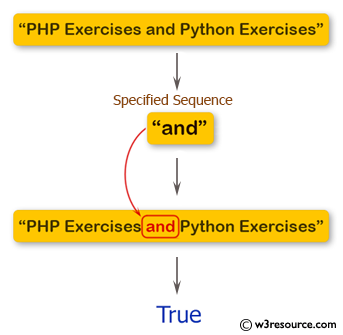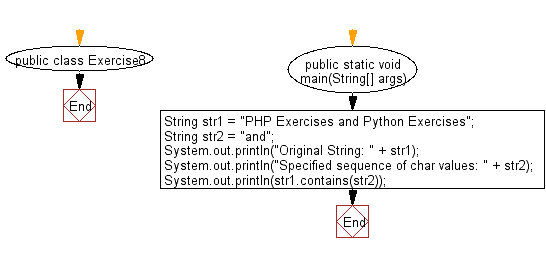﻿ Java exercises: Test if a given string contains the specified sequence of char values - w3resource# Java String Exercises: Test if a given string contains the specified sequence of char values

## Java String: Exercise-8 with Solution

Write a Java program to test if a given string contains the specified sequence of char values.

Pictorial Presentation:Sample Solution:

Java Code:

``````public class Exercise8 {

public static void main(String[] args)
{
String str1 = "PHP Exercises and Python Exercises";
String str2 = "and";
System.out.println("Original String: " + str1);
System.out.println("Specified sequence of char values: " + str2);
System.out.println(str1.contains(str2));
}
}
```
```

Sample Output:

```Original String: PHP Exercises and Python Exercises
Specified sequence of char values: and
true
```

Flowchart:Visualize Java code execution (Python Tutor):

Java Code Editor:

Improve this sample solution and post your code through Disqus

What is the difficulty level of this exercise?

﻿

## Java: Tips of the Day

Parsing dates:

```import java.io.*;
import java.util.*;
import java.text.*;

String s = "2001/09/23 14:39";

SimpleDateFormat formatter = new SimpleDateFormat ("yyyy/MM/dd H:mm");
Date d = formatter.parse(s, new ParsePosition(0));
```